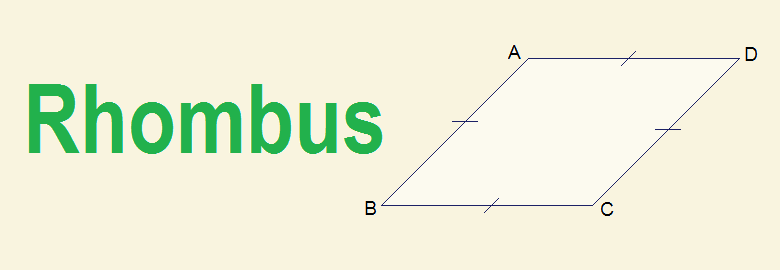# Rhombus | what is Rhombus?## Rhombus | what is Rhombus?

Definition: In geometry, a rhombus is a quadrilateral having its all four sides equal.
In the given figure of quadrilateral ABCD, AB = BC = CD = AD. So ABCD is a rhombus.

Note:
-          A rhombus is also a parallelogram having its all sides equal.
-          Square is a special case of rhombus having all angles 90°.

### Length of a Rhombus

All four sides of a rhombus are equal and the measure of the side is taken as length (l) of the rhombus. In the given figure of rhombus ABCD,
Length of rhombus = AB = BC = CD = AD = l

### Diagonals of a Rhombus

The line joining the opposite vertices of a quadrilateral is called a diagonal. Two diagonals of a rhombus bisect to each other at right angle i.e. 90°.
In the given figure, ABCD is a rhombus. AC and BD are diagonals. Diagonals AC and BD bisect to each other perpendicularly at point O i.e. OA = OC, OB = OD and COD = BOC = AOB = AOD = 90°

### Perimeter and Area of Rhombus

Perimeter and area of a rhombus are given by the formula:

Perimeter = 4l, where l is the length of the side of rhombus.
Area = ½ × d1 × d2, where d1 and d2 two diagonals of rhombus.

### Properties of Rhombus

1.       All sides of a rhombus are equal.
2.       Opposite sides of a rhombus are parallel.
3.       Opposite angles of a rhombus are equal
4.       Diagonals of a rhombus bisect each other at right angle.

You can comment your questions or problems regarding rhombus here.